# Viewing Molecules¶

## Using the MolecularViewer¶

MolecularViewer is a library-agnostic tool to display molecules in chemview. In this section we will see how to use it, and what representations are currently available.

To create a MolecularViewer instance we need the positions of the atoms, as an array of x, y, z coordinates, and a description of the features and connectivity of the system (also called topology).

The topology is a nested dictionary with the following fields:

atom_types

(required field) A list of strings, each representing an atom symbol.

Example: ["H", "C", "N", "O", ..]

bonds

A list of tuples indicating the index of the bond extrema.

Example: [(0, 1), (1, 2), ...]

atom_names

A list of atom names, like the ones used in pdb files

Example: ["HA", "CA", "N", ...]

residue_indices

A nested list of indices (as tuples) for each residue present in the molecule.

Example: [(0, 1, 2, 3, 4, 5), (6, 7, 8, 9, 10), ... ]

residue_types

A list of strings corresponding to residue types.

Example: ["ALA", "GLY", ...]

secondary_structure

A list of strings representing the secondary structure of each residue, H for helix, E for sheet, C for coil.

Example: ["H", "H", "H", "C", "C", "E", "E" ...]

Note

As the description of the topology is quite involved, you can combine chemview with another library that provides the topology directly from the chemical data files (such as chemlab and mdtraj).

Once you create your molecular viewer, you can display the molecule in a variety of ways:

• points: the atomic positions will be represented as points, color-coded by atom.

Example:

mv.points()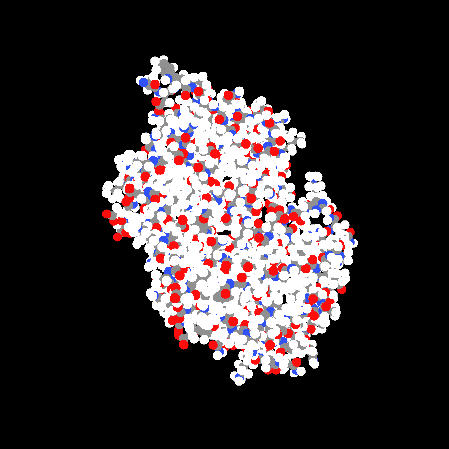• lines: the bonds will be represented as lines

Example:

mv.lines()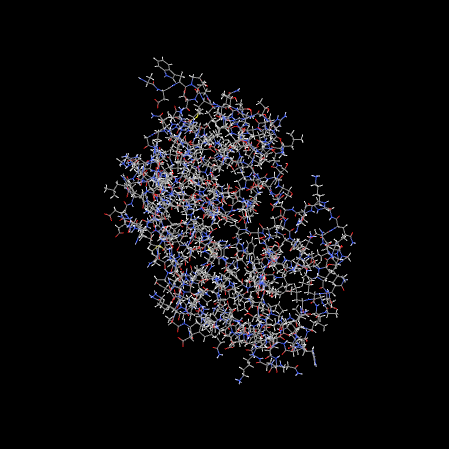• ball_and_sticks: the classical ball and stick representation. Atom are spheres, bonds are cylinders. At the moment this representation is not suitable for very large molecules and animations.

Example:

mv.ball_and_sticks()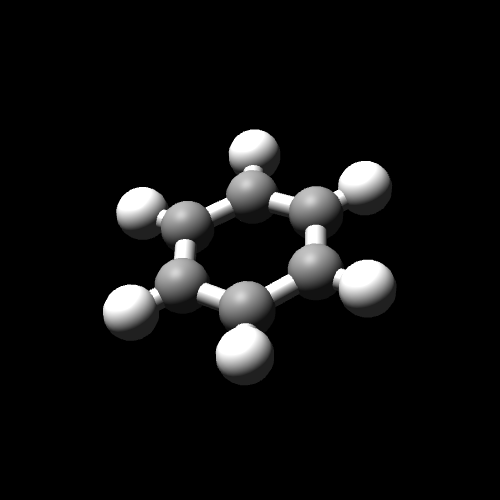• line_ribbon: the protein backbone is represented by a smooth line.

Example:

mv.line_ribbon()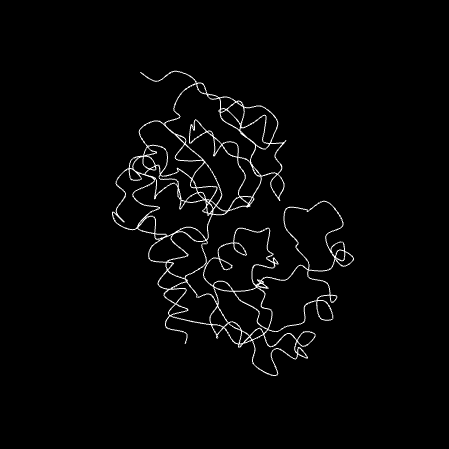• cylinder_and_strand: the protein backbone is represented by a smooth, solid tube, and the helices are represented as cylinders.

Example:

mv.line_ribbon()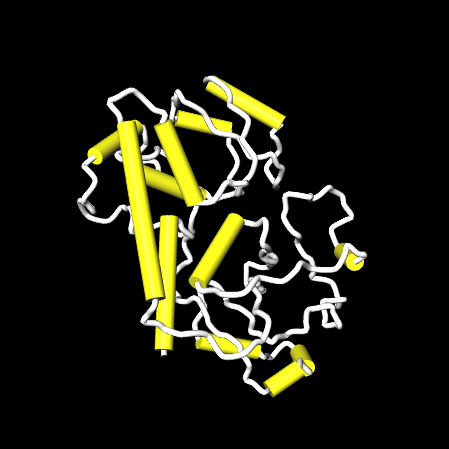The MolecularViewer documentation at api/index

You can also add isosurfaces with the command MolecularViewer.add_isosurface() that takes a function and an isovalue. Given a function $$f(x, y, z)$$, an isosurface is the set of points for which the function assumes a certain value. For example if you want to plot the surface of sphere with radius 1, we can select a function of the type:

$f(x, y, z) = x^2 + y^2 + z^2$

and set the isovalue would be 1, so that we obtain the surface whose set of points that satisfy the equation of a sphere:

$x^2 + y^2 + z^2 = 1$

## Viewing Molecules with Chemlab¶

The development version of chemlab provides a preliminary integration with chemview, check out the example notebook.

## Viewing Molecules with MDTraj¶

In the near future, mdtraj will provide integration.

While you wait, take a look at the docs and learn about mdtraj.

## Making custom representations¶

chemview provides an easy-to-use API to create new ways to display your data and build novel tools. The class RepresentationViewer contains methods to display common 3D shapes.

To create a RepresentationViewer instance, type:

rv = RepresentationViewer()
rv


This will display an empty viewer. To add objects, we can use the method RepresentationViewer.add_representation(). The method takes two parameters: the name of the representation to display, and a dictionary of options, that are specific for each representation.

For example, to add three points on the screen we will use the following parameters:

rv.add_representation('points', {'coordinates', np.array([[0.0, 0.0, 0.0],
1.0, 0.0, 0.0],
2.0, 0.0, 0.0])})


Warning

The RepresentationViewer communicates directly with the Javascript layer and, being outside of the realm of Python doesn’t provide nice exception tracebacks. Be rigorous with parameter types.

For more examples (with pictures) you can check the test notebook.

Below reference of the available representations, along with their options:

points

display a set of coordinates as points with different colors and sizes.

Options:

• coordinates

numpy array of 3D coordinates (float32)

• sizes

python list of floats representing the size of each point

• colors

python list of 32 bit integers representing the color of each point.

Example using HEX representation: [0xffffff, 0x00ffff, 0xff0000, ...]

lines

display a set of lines with different colors.

Options:

• startCoords
numpy array of 3D coordinates representing the starting point of each line
• endCoords
numpy array of 3D coordinates representing the ending point of each line
• startColors
list of 32 bit integers corresponding to the color of the starting point
• endColors
list of 32 bit integers corresponding to the color of the ending point
cylinders

display a set of cylinders. This is a slow primitive, avoid using it for animations; use lines instead.

Options:

• startCoords
numpy array of 3D coordinates representing the starting point of each cylinder
• endCoords
numpy array of 3D coordinates representing the ending point of each cylinder
• colors
list of 32 bit integers corresponding to the color of each cylinder
list of float corresponding to the radius of each cylinder
smoothline

display a smooth line that passes through a set of points.

Options:

• coordinates
numpy array of 3D coordinates representing the control points of the smooth line.
• color
32 bit integer (hex) color of the line
• resolution
int, number of subdivision along the path between control points. Controls the smoothness
smoothtube

display a smooth tube that passes through a set of points. This is a slow primitive, not suitable for animating very large objects; use smoothline instead.

Options:

• coordinates
numpy array of 3D coordinates representing the control points of the smooth tube.
• color
32 bit integer (hex) color of the tube
float representing the radius of the tube
• resolution
int, number of subdivision along the path between control points. Controls the smoothness
spheres

display a set of spheres. This primitive is slow, avoid using it for animations; use points instead.

Options:

• coordinates
numpy array of 3D coordinates representing the position of the spheres.
• colors
list of 32 bit integers representing the color of each sphere